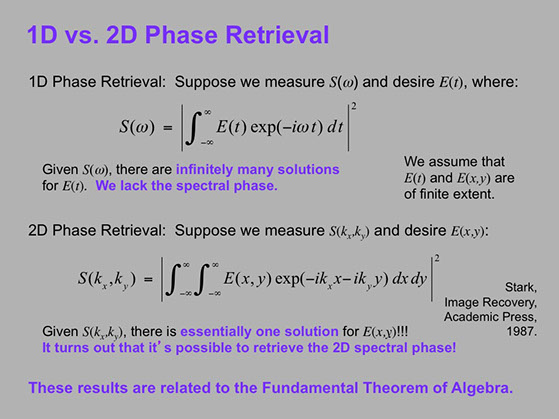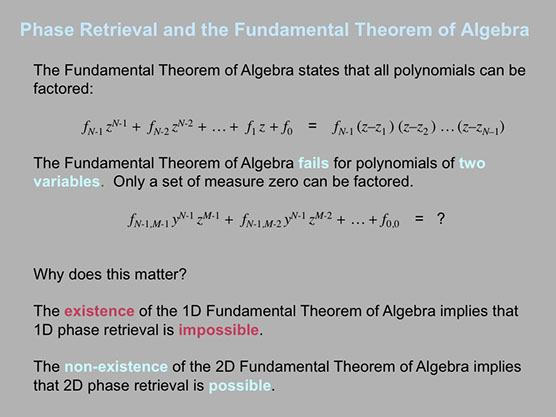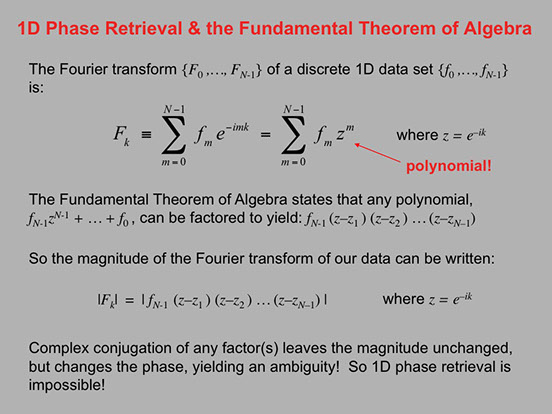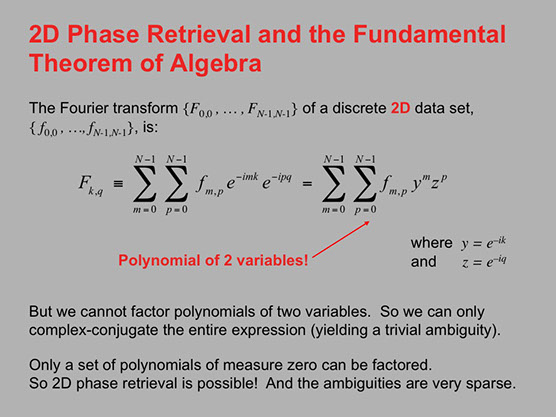Now that sounds massively unintuitive!  Let's explain this in a bit more detail!

Let's begin by stating the problem more precisely mathematically.

Here are the precise statements of the 1D and 2D Phase Retrieval problems.And let's state precisely the Fundamental Theorems of Algebra for polynomials of one and two variables.

In this analysis, all quantities (in particular, Fk and fk below) are potentially complex numbers and so have real and imaginary parts, or equivalently, intensity and phase.

Now since data are always discrete (not continuous), we need to use the discrete Fourier transform. So imagine that we measure the magnitudes of the set of Fk. And we're trying to find the set of fk (that is, phase retrieval). But we can factor the resulting polynomial, and complex-conjugating the resulting factors generates additional solutions--i.e., ambiguities!Because the Fundamental Theorem of Algebra fails for polynomials of two variables, factoring the polynomial in y and z isn't possible!  So we can't complex-conjugate factors to generate ambiguities, and 2D Phase Retrieval yields a unique solution (except for very very rare cases when the polynomial, which is typically of very high order, can actually be factored).

Prev

Less

Next